# Maths in daily life

15 Nov 2013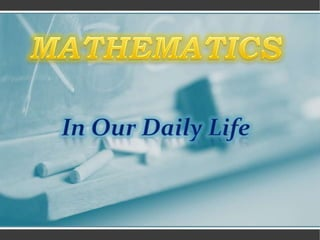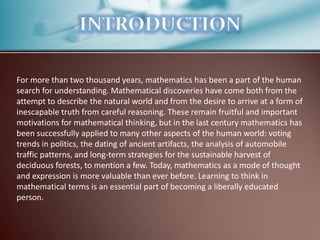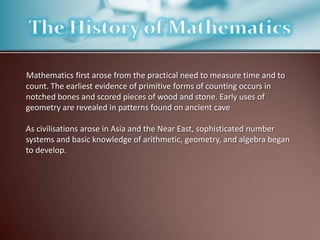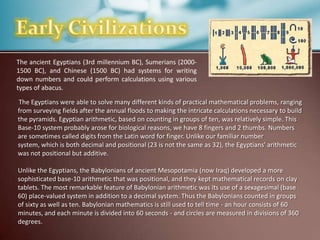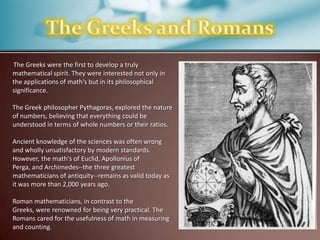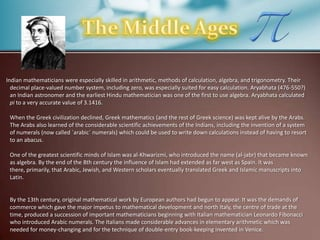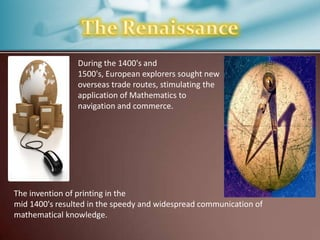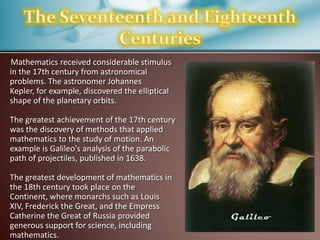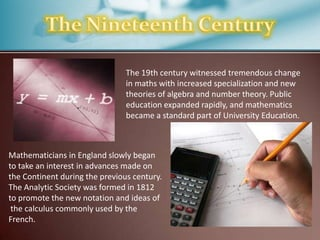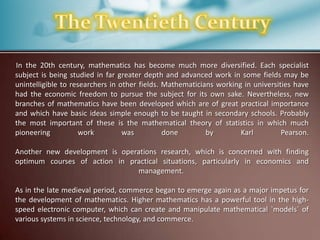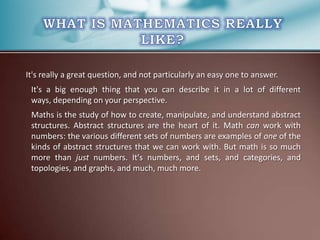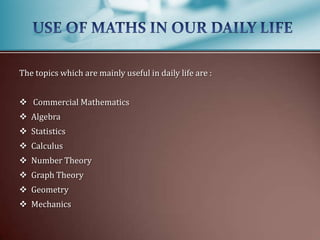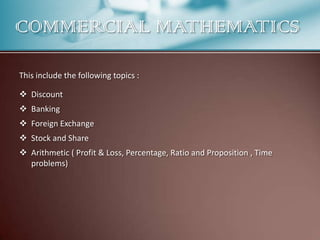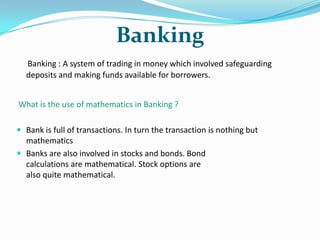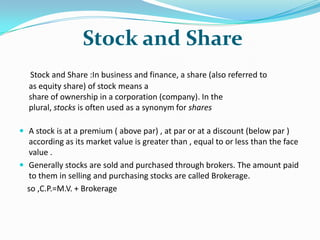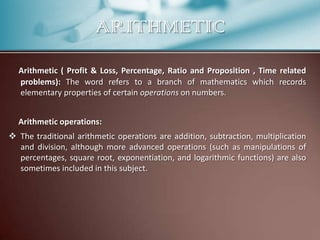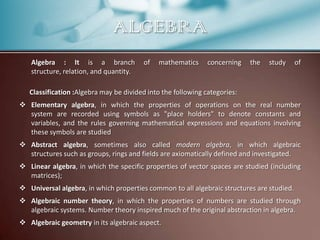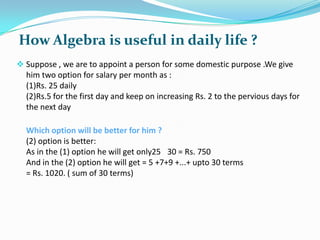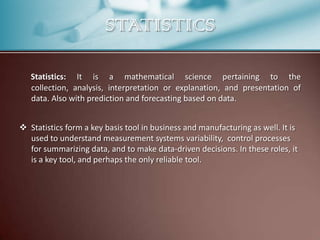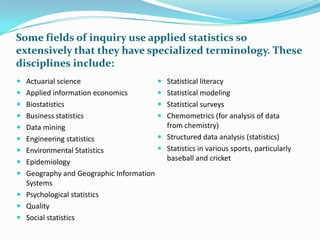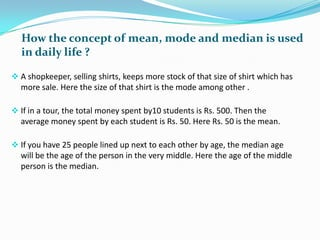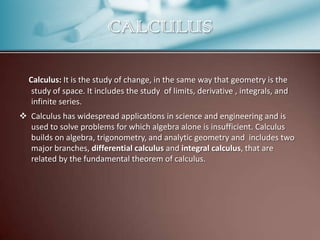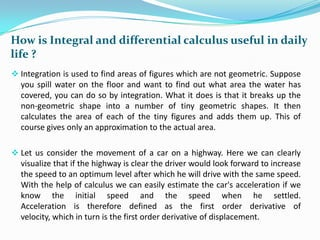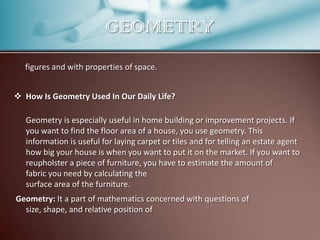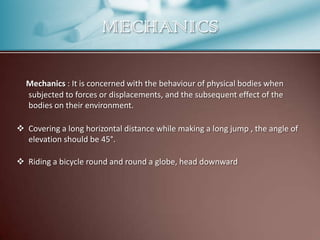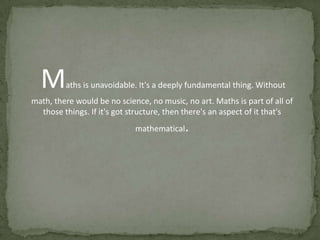1 sur 29

### Maths in daily life

• 2. For more than two thousand years, mathematics has been a part of the human search for understanding. Mathematical discoveries have come both from the attempt to describe the natural world and from the desire to arrive at a form of inescapable truth from careful reasoning. These remain fruitful and important motivations for mathematical thinking, but in the last century mathematics has been successfully applied to many other aspects of the human world: voting trends in politics, the dating of ancient artifacts, the analysis of automobile traffic patterns, and long-term strategies for the sustainable harvest of deciduous forests, to mention a few. Today, mathematics as a mode of thought and expression is more valuable than ever before. Learning to think in mathematical terms is an essential part of becoming a liberally educated person.
• 3. Mathematics first arose from the practical need to measure time and to count. The earliest evidence of primitive forms of counting occurs in notched bones and scored pieces of wood and stone. Early uses of geometry are revealed in patterns found on ancient cave As civilisations arose in Asia and the Near East, sophisticated number systems and basic knowledge of arithmetic, geometry, and algebra began to develop.
• 4. The ancient Egyptians (3rd millennium BC), Sumerians (20001500 BC), and Chinese (1500 BC) had systems for writing down numbers and could perform calculations using various types of abacus. The Egyptians were able to solve many different kinds of practical mathematical problems, ranging from surveying fields after the annual floods to making the intricate calculations necessary to build the pyramids. Egyptian arithmetic, based on counting in groups of ten, was relatively simple. This Base-10 system probably arose for biological reasons, we have 8 fingers and 2 thumbs. Numbers are sometimes called digits from the Latin word for finger. Unlike our familiar number system, which is both decimal and positional (23 is not the same as 32), the Egyptians' arithmetic was not positional but additive. Unlike the Egyptians, the Babylonians of ancient Mesopotamia (now Iraq) developed a more sophisticated base-10 arithmetic that was positional, and they kept mathematical records on clay tablets. The most remarkable feature of Babylonian arithmetic was its use of a sexagesimal (base 60) place-valued system in addition to a decimal system. Thus the Babylonians counted in groups of sixty as well as ten. Babylonian mathematics is still used to tell time - an hour consists of 60 minutes, and each minute is divided into 60 seconds - and circles are measured in divisions of 360 degrees.
• 5. The Greeks were the first to develop a truly mathematical spirit. They were interested not only in the applications of math's but in its philosophical significance. The Greek philosopher Pythagoras, explored the nature of numbers, believing that everything could be understood in terms of whole numbers or their ratios. Ancient knowledge of the sciences was often wrong and wholly unsatisfactory by modern standards. However, the math's of Euclid, Apollonius of Perga, and Archimedes--the three greatest mathematicians of antiquity--remains as valid today as it was more than 2,000 years ago. Roman mathematicians, in contrast to the Greeks, were renowned for being very practical. The Romans cared for the usefulness of math in measuring and counting.
• 6. Indian mathematicians were especially skilled in arithmetic, methods of calculation, algebra, and trigonometry. Their decimal place-valued number system, including zero, was especially suited for easy calculation. Aryabhata (476-550?) an Indian astronomer and the earliest Hindu mathematician was one of the first to use algebra. Aryabhata calculated pi to a very accurate value of 3.1416. When the Greek civilization declined, Greek mathematics (and the rest of Greek science) was kept alive by the Arabs. The Arabs also learned of the considerable scientific achievements of the Indians, including the invention of a system of numerals (now called `arabic´ numerals) which could be used to write down calculations instead of having to resort to an abacus. One of the greatest scientific minds of Islam was al-Khwarizmi, who introduced the name (al-jabr) that became known as algebra. By the end of the 8th century the influence of Islam had extended as far west as Spain. It was there, primarily, that Arabic, Jewish, and Western scholars eventually translated Greek and Islamic manuscripts into Latin. By the 13th century, original mathematical work by European authors had begun to appear. It was the demands of commerce which gave the major impetus to mathematical development and north Italy, the centre of trade at the time, produced a succession of important mathematicians beginning with Italian mathematician Leonardo Fibonacci who introduced Arabic numerals. The Italians made considerable advances in elementary arithmetic which was needed for money-changing and for the technique of double-entry book-keeping invented in Venice.
• 7. During the 1400's and 1500's, European explorers sought new overseas trade routes, stimulating the application of Mathematics to navigation and commerce. The invention of printing in the mid 1400's resulted in the speedy and widespread communication of mathematical knowledge.
• 8. Mathematics received considerable stimulus in the 17th century from astronomical problems. The astronomer Johannes Kepler, for example, discovered the elliptical shape of the planetary orbits. The greatest achievement of the 17th century was the discovery of methods that applied mathematics to the study of motion. An example is Galileo's analysis of the parabolic path of projectiles, published in 1638. The greatest development of mathematics in the 18th century took place on the Continent, where monarchs such as Louis XIV, Frederick the Great, and the Empress Catherine the Great of Russia provided generous support for science, including mathematics.
• 9. The 19th century witnessed tremendous change in maths with increased specialization and new theories of algebra and number theory. Public education expanded rapidly, and mathematics became a standard part of University Education. Mathematicians in England slowly began to take an interest in advances made on the Continent during the previous century. The Analytic Society was formed in 1812 to promote the new notation and ideas of the calculus commonly used by the French.
• 10. In the 20th century, mathematics has become much more diversified. Each specialist subject is being studied in far greater depth and advanced work in some fields may be unintelligible to researchers in other fields. Mathematicians working in universities have had the economic freedom to pursue the subject for its own sake. Nevertheless, new branches of mathematics have been developed which are of great practical importance and which have basic ideas simple enough to be taught in secondary schools. Probably the most important of these is the mathematical theory of statistics in which much pioneering work was done by Karl Pearson. Another new development is operations research, which is concerned with finding optimum courses of action in practical situations, particularly in economics and management. As in the late medieval period, commerce began to emerge again as a major impetus for the development of mathematics. Higher mathematics has a powerful tool in the highspeed electronic computer, which can create and manipulate mathematical `models´ of various systems in science, technology, and commerce.
• 11. It's really a great question, and not particularly an easy one to answer. It's a big enough thing that you can describe it in a lot of different ways, depending on your perspective. Maths is the study of how to create, manipulate, and understand abstract structures. Abstract structures are the heart of it. Math can work with numbers: the various different sets of numbers are examples of one of the kinds of abstract structures that we can work with. But math is so much more than just numbers. It's numbers, and sets, and categories, and topologies, and graphs, and much, much more.
• 12. The topics which are mainly useful in daily life are :  Commercial Mathematics  Algebra  Statistics  Calculus  Number Theory  Graph Theory  Geometry  Mechanics
• 13. COMMERCIAL MATHEMATICS This include the following topics :  Discount  Banking  Foreign Exchange  Stock and Share  Arithmetic ( Profit & Loss, Percentage, Ratio and Proposition , Time problems)
• 15. Banking Banking : A system of trading in money which involved safeguarding deposits and making funds available for borrowers. What is the use of mathematics in Banking ?  Bank is full of transactions. In turn the transaction is nothing but mathematics  Banks are also involved in stocks and bonds. Bond calculations are mathematical. Stock options are also quite mathematical.
• 16. Foreign Exchange Market The foreign exchange (currency) market refers to the market for currencies. Transactions in this market typically involve one party purchasing a quantity of one currency in exchange for paying a quantity of another.  What are the rate of exchange of currencies of different counties w.r.t. Indian currencies?
• 17. Stock and Share Stock and Share :In business and finance, a share (also referred to as equity share) of stock means a share of ownership in a corporation (company). In the plural, stocks is often used as a synonym for shares  A stock is at a premium ( above par) , at par or at a discount (below par ) according as its market value is greater than , equal to or less than the face value .  Generally stocks are sold and purchased through brokers. The amount paid to them in selling and purchasing stocks are called Brokerage. so ,C.P.=M.V. + Brokerage
• 18. ARITHMETIC Arithmetic ( Profit & Loss, Percentage, Ratio and Proposition , Time related problems): The word refers to a branch of mathematics which records elementary properties of certain operations on numbers. Arithmetic operations:  The traditional arithmetic operations are addition, subtraction, multiplication and division, although more advanced operations (such as manipulations of percentages, square root, exponentiation, and logarithmic functions) are also sometimes included in this subject.
• 19. ALGEBRA Algebra : It is a branch structure, relation, and quantity. of mathematics concerning the study of Classification :Algebra may be divided into the following categories:  Elementary algebra, in which the properties of operations on the real number system are recorded using symbols as "place holders" to denote constants and variables, and the rules governing mathematical expressions and equations involving these symbols are studied  Abstract algebra, sometimes also called modern algebra, in which algebraic structures such as groups, rings and fields are axiomatically defined and investigated.  Linear algebra, in which the specific properties of vector spaces are studied (including matrices);  Universal algebra, in which properties common to all algebraic structures are studied.  Algebraic number theory, in which the properties of numbers are studied through algebraic systems. Number theory inspired much of the original abstraction in algebra.  Algebraic geometry in its algebraic aspect.
• 20. How Algebra is useful in daily life ?  Suppose , we are to appoint a person for some domestic purpose .We give him two option for salary per month as : (1)Rs. 25 daily (2)Rs.5 for the first day and keep on increasing Rs. 2 to the pervious days for the next day Which option will be better for him ? (2) option is better: As in the (1) option he will get only25 30 = Rs. 750 And in the (2) option he will get = 5 +7+9 +...+ upto 30 terms = Rs. 1020. ( sum of 30 terms)
• 21. STATISTICS Statistics: It is a mathematical science pertaining to the collection, analysis, interpretation or explanation, and presentation of data. Also with prediction and forecasting based on data.  Statistics form a key basis tool in business and manufacturing as well. It is used to understand measurement systems variability, control processes for summarizing data, and to make data-driven decisions. In these roles, it is a key tool, and perhaps the only reliable tool.
• 22. Some fields of inquiry use applied statistics so extensively that they have specialized terminology. These disciplines include:  Actuarial science  Statistical literacy  Applied information economics  Statistical modeling  Biostatistics  Statistical surveys  Business statistics  Data mining  Chemometrics (for analysis of data from chemistry)  Engineering statistics  Structured data analysis (statistics)  Environmental Statistics  Statistics in various sports, particularly baseball and cricket  Epidemiology  Geography and Geographic Information Systems  Psychological statistics  Quality  Social statistics
• 23. How the concept of mean, mode and median is used in daily life ?  A shopkeeper, selling shirts, keeps more stock of that size of shirt which has more sale. Here the size of that shirt is the mode among other .  If in a tour, the total money spent by10 students is Rs. 500. Then the average money spent by each student is Rs. 50. Here Rs. 50 is the mean.  If you have 25 people lined up next to each other by age, the median age will be the age of the person in the very middle. Here the age of the middle person is the median.
• 24. CALCULUS Calculus: It is the study of change, in the same way that geometry is the study of space. It includes the study of limits, derivative , integrals, and infinite series.  Calculus has widespread applications in science and engineering and is used to solve problems for which algebra alone is insufficient. Calculus builds on algebra, trigonometry, and analytic geometry and includes two major branches, differential calculus and integral calculus, that are related by the fundamental theorem of calculus.
• 25. How is Integral and differential calculus useful in daily life ?  Integration is used to find areas of figures which are not geometric. Suppose you spill water on the floor and want to find out what area the water has covered, you can do so by integration. What it does is that it breaks up the non-geometric shape into a number of tiny geometric shapes. It then calculates the area of each of the tiny figures and adds them up. This of course gives only an approximation to the actual area.  Let us consider the movement of a car on a highway. Here we can clearly visualize that if the highway is clear the driver would look forward to increase the speed to an optimum level after which he will drive with the same speed. With the help of calculus we can easily estimate the car's acceleration if we know the initial speed and the speed when he settled. Acceleration is therefore defined as the first order derivative of velocity, which in turn is the first order derivative of displacement.
• 26. GEOMETRY figures and with properties of space.  How Is Geometry Used In Our Daily Life? Geometry is especially useful in home building or improvement projects. If you want to find the floor area of a house, you use geometry. This information is useful for laying carpet or tiles and for telling an estate agent how big your house is when you want to put it on the market. If you want to reupholster a piece of furniture, you have to estimate the amount of fabric you need by calculating the surface area of the furniture. Geometry: It a part of mathematics concerned with questions of size, shape, and relative position of
• 27. MECHANICS Mechanics : It is concerned with the behaviour of physical bodies when subjected to forces or displacements, and the subsequent effect of the bodies on their environment.  Covering a long horizontal distance while making a long jump , the angle of elevation should be 45°.  Riding a bicycle round and round a globe, head downward
• 28. M aths is unavoidable. It's a deeply fundamental thing. Without math, there would be no science, no music, no art. Maths is part of all of those things. If it's got structure, then there's an aspect of it that's . mathematical